Anúncio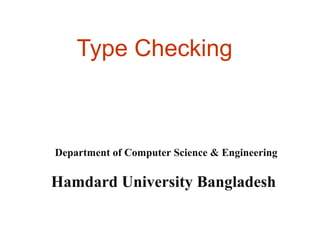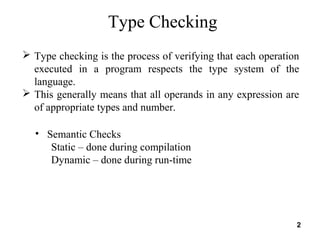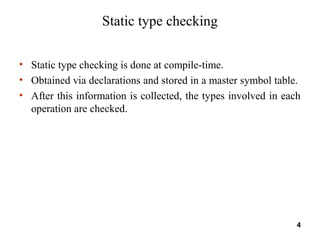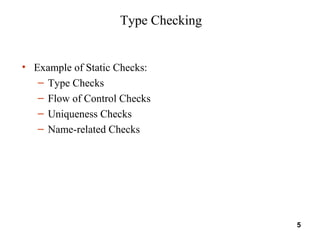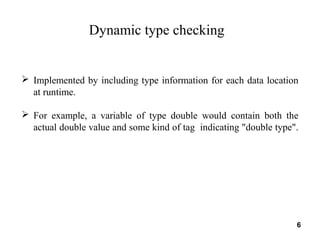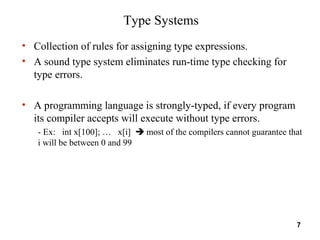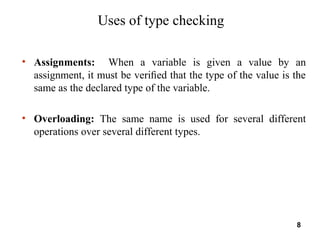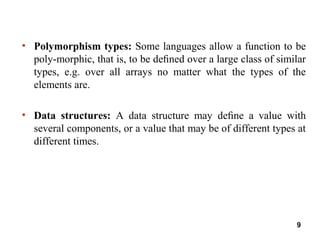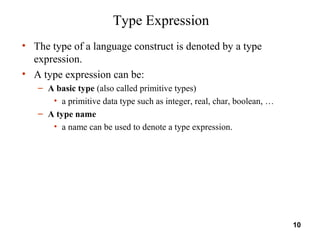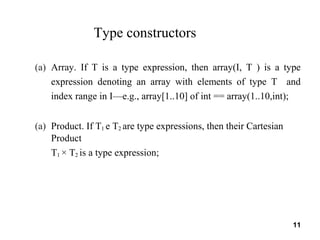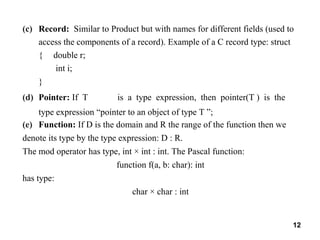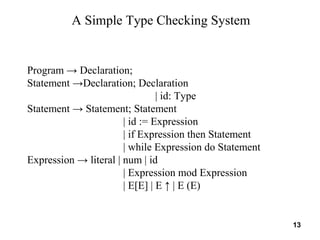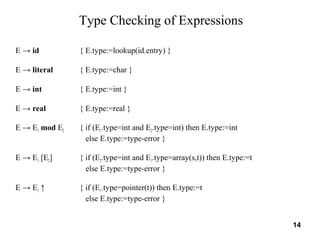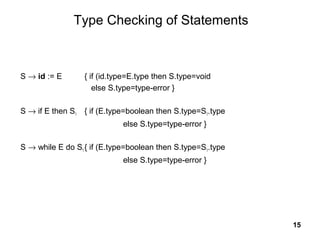1 de 15
Anúncio

### Type Checking(Compiler Design) #ShareThisIfYouLike

1. Type Checking Department of Computer Science & Engineering Hamdard University Bangladesh
2.  Type checking is the process of verifying that each operation executed in a program respects the type system of the language.  This generally means that all operands in any expression are of appropriate types and number. • Semantic Checks Static – done during compilation Dynamic – done during run-time Type Checking 2
3. 1.Identify the types that are available in the language 2.Identify the language constructs that have types associated with them 3. Identify the semantic rules for the language Process of designing a type checker 3
4. Static type checking • Static type checking is done at compile-time. • Obtained via declarations and stored in a master symbol table. • After this information is collected, the types involved in each operation are checked. 4
5. Type Checking • Example of Static Checks: – Type Checks – Flow of Control Checks – Uniqueness Checks – Name-related Checks 5
6.  Implemented by including type information for each data location at runtime.  For example, a variable of type double would contain both the actual double value and some kind of tag indicating "double type". Dynamic type checking 6
7. Type Systems • Collection of rules for assigning type expressions. • A sound type system eliminates run-time type checking for type errors. • A programming language is strongly-typed, if every program its compiler accepts will execute without type errors. - Ex: int x; … x[i]  most of the compilers cannot guarantee that i will be between 0 and 99 7
8. Uses of type checking • Assignments: When a variable is given a value by an assignment, it must be veriﬁed that the type of the value is the same as the declared type of the variable. • Overloading: The same name is used for several different operations over several different types. 8
9. • Polymorphism types: Some languages allow a function to be poly-morphic, that is, to be deﬁned over a large class of similar types, e.g. over all arrays no matter what the types of the elements are. • Data structures: A data structure may deﬁne a value with several components, or a value that may be of different types at different times. 9
10. Type Expression • The type of a language construct is denoted by a type expression. • A type expression can be: – A basic type (also called primitive types) • a primitive data type such as integer, real, char, boolean, … – A type name • a name can be used to denote a type expression. 10
11. (a) Array. If T is a type expression, then array(I, T ) is a type expression denoting an array with elements of type T and index range in I—e.g., array[1..10] of int == array(1..10,int); (a) Product. If T1 e T2 are type expressions, then their Cartesian Product T1 × T2 is a type expression; Type constructors 11
12. (c) Record: Similar to Product but with names for different fields (used to access the components of a record). Example of a C record type: struct { double r; int i; } (d) Pointer: If T is a type expression, then pointer(T ) is the type expression “pointer to an object of type T ”; (e) Function: If D is the domain and R the range of the function then we denote its type by the type expression: D : R. The mod operator has type, int × int : int. The Pascal function: function f(a, b: char): int has type: char × char : int 12
13. A Simple Type Checking System Program → Declaration; Statement →Declaration; Declaration | id: Type Statement → Statement; Statement | id := Expression | if Expression then Statement | while Expression do Statement Expression → literal | num | id | Expression mod Expression | E[E] | E ↑ | E (E) 13
14. Type Checking of Expressions E → id { E.type:=lookup(id.entry) } E → literal { E.type:=char } E → int { E.type:=int } E → real { E.type:=real } E → E1 mod E2 { if (E1.type=int and E2.type=int) then E.type:=int else E.type:=type-error } E → E1 [E2] { if (E2.type=int and E1.type=array(s,t)) then E.type:=t else E.type:=type-error } E → E1 ↑ { if (E1.type=pointer(t)) then E.type:=t else E.type:=type-error } 14
15. Type Checking of Statements S → id := E { if (id.type=E.type then S.type=void else S.type=type-error } S → if E then S1 { if (E.type=boolean then S.type=S1.type else S.type=type-error } S → while E do S1{ if (E.type=boolean then S.type=S1.type else S.type=type-error } 15
Anúncio